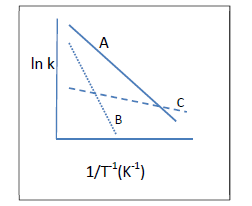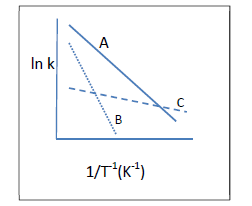# Problem: Each line in the graph below represents a different reaction. List the reactions in order of increasing activation energy.A) A&lt; B &lt; CB) C &lt; A &lt; BC) B &lt; A &lt; CD) C &lt; B &lt; AE) this graph is only for first order rxns

###### FREE Expert Solution

We’re being asked to list the given reactions in order of increasing activation energy based on the given plots:We’re given the plot of ln k (y) vs. 1/T (x)

This means we need to use the two-point form of the Arrhenius Equation:

where:

k = rate constant

Ea = activation energy (in J/mol)

R = gas constant (8.314 J/mol • K)

T = temperature (in K)

A = Arrhenius constant or frequency factor###### Problem Details

Each line in the graph below represents a different reaction. List the reactions in order of increasing activation energy.

A) A< B < C
B) C < A < B
C) B < A < C
D) C < B < A
E) this graph is only for first order rxnsWhat scientific concept do you need to know in order to solve this problem?

Our tutors have indicated that to solve this problem you will need to apply the Arrhenius Equation concept. You can view video lessons to learn Arrhenius Equation. Or if you need more Arrhenius Equation practice, you can also practice Arrhenius Equation practice problems.

What is the difficulty of this problem?

Our tutors rated the difficulty ofEach line in the graph below represents a different reaction...as medium difficulty.

How long does this problem take to solve?

Our expert Chemistry tutor, Jules took 3 minutes and 6 seconds to solve this problem. You can follow their steps in the video explanation above.

What professor is this problem relevant for?

Based on our data, we think this problem is relevant for Professor Becker's class at FIU.